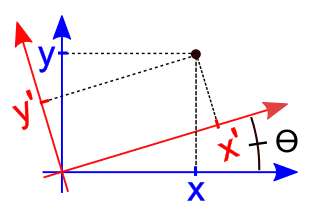# Rotation of axesAn xy-Cartesian coordinate system rotated through an angleto an x'y'-Cartesian coordinate system

In mathematics, a rotation of axes in two dimensions is a mapping from an xy-Cartesian coordinate system to an x'y'-Cartesian coordinate system in which the origin is kept fixed and the x' and y' axes are obtained by rotating the x and y axes counterclockwise through an angle. A point P has coordinates (x, y) with respect to the original system and coordinates (x', y') with respect to the new system. In the new coordinate system, the point P will appear to have been rotated in the opposite direction, that is, clockwise through the angle. A rotation of axes in more than two dimensions is defined similarly. A rotation of axes is a linear map and a rigid transformation.

## Motivation

Coordinate systems are essential for studying the equations of curves using the methods of analytic geometry. To use the method of coordinate geometry, the axes are placed at a convenient position with respect to the curve under consideration. For example, to study the equations of ellipses and hyperbolas, the foci are usually located on one of the axes and are situated symmetrically with respect to the origin. If the curve (hyperbola, parabola, ellipse, etc.) is not situated conveniently with respect to the axes, the coordinate system should be changed to place the curve at a convenient and familiar location and orientation. The process of making this change is called a transformation of coordinates.

The solutions to many problems can be simplified by rotating the coordinate axes to obtain new axes through the same origin.

## Derivation

The equations defining the transformation in two dimensions, which rotates the xy axes counterclockwise through an angleinto the x'y' axes, are derived as follows.

In the xy system, let the point P have polar coordinates. Then, in the x'y' system, P will have polar coordinates.

We have(1)(2)

and(3)(4)

Substituting equations (1) and (2) into equations (3) and (4), we obtain(5)(6)

Equations (5) and (6) can be represented in matrix form aswhich is the standard matrix equation of a rotation of axes in two dimensions.

The inverse transformation is(7)(8)

or## Examples in two dimensions

### Example 1

Find the coordinates of the pointafter the axes have been rotated through the angle, or 30°.

Solution:The axes have been rotated counterclockwise through an angle ofand the new coordinates are. Note that the point appears to have been rotated clockwise through, that is, it now coincides with the (new) x' axis.

### Example 2

Find the coordinates of the pointafter the axes have been rotated clockwise 90°, that is, through the angle, or -90°.

Solution:The axes have been rotated through an angle of, which is in the clockwise direction and the new coordinates are. Again, note that the point appears to have been rotated counterclockwise through.

## Rotation of conic sections

Main article: Conic section

The most general equation of the second degree has the form(not all zero).

(9)

Through a change of coordinates (a rotation of axes and a translation of axes), equation (9) can be put into a standard form, which is usually easier to work with. It is always possible to rotate the coordinates in such a way that in the new system there is no x'y' term. Substituting equations (7) and (8) into equation (9), we obtain(10)

where(11)

Ifis selected so thatwe will haveand the x'y' term in equation (10) will vanish.

When a problem arises with B, D and E all different from zero, they can be eliminated by performing in succession a rotation (eliminating B) and a translation (eliminating the D and E terms).

### Identifying rotated conic sections

A non-degenerate conic section given by equation (9) can be identified by evaluating. The conic section is:## Generalization to several dimensions

Suppose a rectangular xyz-coordinate system is rotated around its z axis counterclockwise (looking down the positive z axis) through an angle, that is, the positive x axis is rotated immediately into the positive y axis. The z coordinate of each point is unchanged and the x and y coordinates transform as above. The old coordinates (x, y, z) of a point Q are related to its new coordinates (x', y', z') byGeneralizing to any finite number of dimensions, a rotation matrixis an orthogonal matrix that differs from the identity matrix in at most four elements. These four elements are of the formandfor someand some ij.

## Examples in several dimensions

### Example 3

Find the coordinates of the pointafter the positive w axis has been rotated through the angle, or 15°, into the positive z axis.

Solution:## Notes

1. Protter & Morrey (1970, p. 320)
2. Anton (1987, p. 231)
3. Burden & Faires (1993, p. 532)
4. Anton (1987, p. 247)
5. Beauregard & Fraleigh (1973, p. 266)
6. Protter & Morrey (1970, pp. 314-315)
7. Protter & Morrey (1970, pp. 320-321)
8. Anton (1987, p. 230)
9. Protter & Morrey (1970, p. 320)
10. Protter & Morrey (1970, p. 316)
11. Protter & Morrey (1970, pp. 321-322)
12. Protter & Morrey (1970, p. 324)
13. Protter & Morrey (1970, p. 326)
14. Anton (1987, p. 231)
15. Burden & Faires (1993, p. 532)

## References

• Anton, Howard (1987), Elementary Linear Algebra (5th ed.), New York: Wiley, ISBN 0-471-84819-0
• Beauregard, Raymond A.; Fraleigh, John B. (1973), A First Course In Linear Algebra: with Optional Introduction to Groups, Rings, and Fields, Boston: Houghton Mifflin Co., ISBN 0-395-14017-X
• Burden, Richard L.; Faires, J. Douglas (1993), Numerical Analysis (5th ed.), Boston: Prindle, Weber and Schmidt, ISBN 0-534-93219-3
• Protter, Murray H.; Morrey, Jr., Charles B. (1970), College Calculus with Analytic Geometry (2nd ed.), Reading: Addison-Wesley, LCCN 76087042